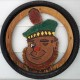# Elucidation

One of my first postings was called A bit of humor. Here is why I like that posting.

We see the decimal numbering system daily. Our minds are trained from infancy to see things from the decimal perspective. That is why we immediately assign values to the digits we see. We even say the names of the values to ourselves in our minds, and to ourselves and others when we read aloud. The mind typically sees the digits 10 and excludes all non decimal interpretations of these digits. It isn’t that the other interpretations don’t exist. It is just that we live in a decimal oriented world.

In the binary world, things are just a little bit different. Since the only recognized digits in binary are 0 and 1, it is necessary to represent higher values in some other (non decimal) way. The following is a comparison of the decimal and binary representation of the numbers from zero to three.

Decimal   Binary

0 = 0

1 = 1

2 = 10

3 = 11

Now you can see why there are only 10 (binary) kinds of people.

1.Huh?

•I couldn’t tell if you were serious about the ‘Huh?’ so I’ll answer as if you really wanted a better explanation.

The decimal number system includes ten digits, but ten is not one of them. The digits are 0, 1, 2, 3, 4, 5, 6, 7, 8, and 9. Numbers in the decimal numbering system use a column for units, a column for tens (bases) a column for tens of tens (base squared) a column for tens of tens of tens (base to the third power) etc. In decimal, when we have ten, we write it as one (1) ten and zero (0) units, or 10.

Binary works the same way.

The binary number system includes two digits, but two is not one of them. The digits are 0 and 1. Numbers in the binary numbering system use a column for units, a column for twos (bases) a column for twos of twos (base squared) a column for twos of twos of twos (base to the third power) etc. In binary, when we have two, we write it as one (1) two and zero (0) units, or 10. The decimal ten and the binary two look the same, but they represent different values.